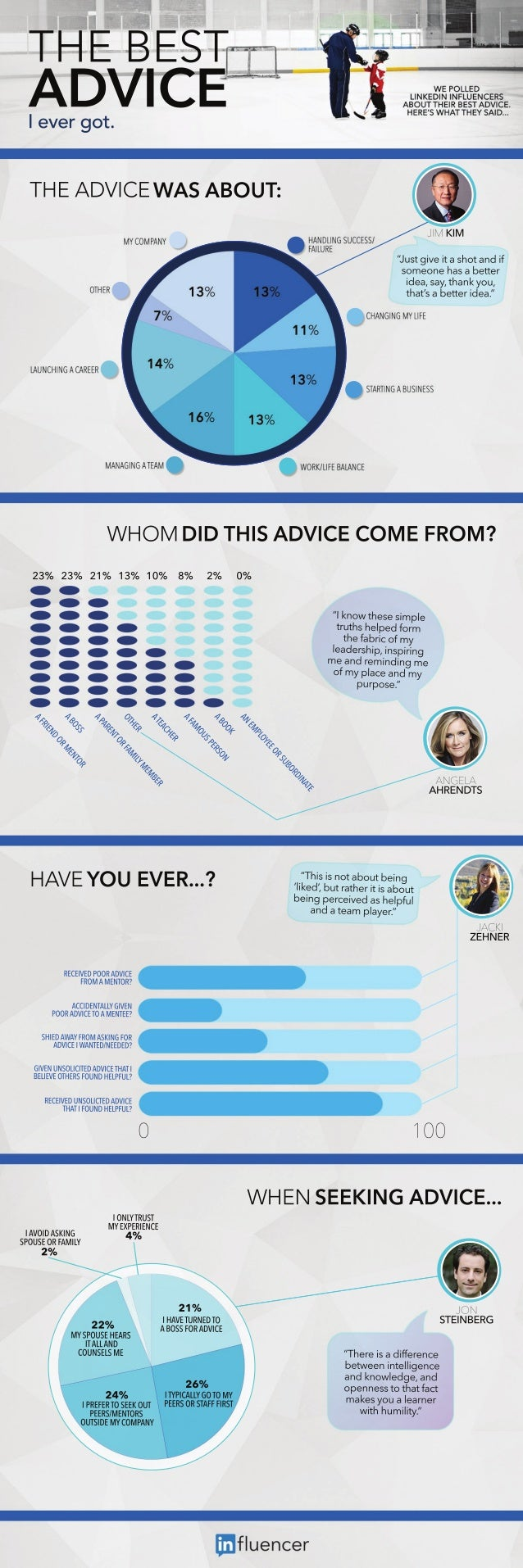Successfully reported this slideshow.
We use your LinkedIn profile and activity data to personalize ads and to show you more relevant ads. You can change your ad preferences anytime.Upcoming SlideShare
×

LinkedIn Influencers Share the Best Advice They Ever Got

36,078 views

Published on

We polled LinkedIn Influencers about the best advice they've ever received. Here's what they said...

Published in: Career
• Full Name
Comment goes here.

Are you sure you want to Yes No
Your message goes here• LinkdeIn shares the best influencers of e-book on the pages of cultures of the world we always reflect out our best ways of digital media and professional technologies of communication worldwide..

Are you sure you want to  Yes  No
Your message goes here

LinkedIn Influencers Share the Best Advice They Ever Got

1. 1. T EB S H ET A VC D IE I vr o. ee g t T EA VC WA A OU : H D IE S B T J KM I I M “ sgv i so a di J t i t h tn f u e a sme n h s b t r o o e a a et e ie ,a, a k o , d asyt n y u h ta’ b t r e . h t a et i a s ed ” WH OM D DT I A VC C I HS D IE OMEF OM? R 2% 2% 2% 1% 1% 8 3 3 1 3 0 % 2 % 0 % “k o tee i l In w h s s e mp t ts e e fr r h h l d om u p te a r o my hf i f bc l d r i, si g e es pi pr a h n i n me n rmi i me ad e n n dg o my l e n my f pa a d c p roe up s. ” E T A N I D R O B U S R O E E Y O L MP E N A K O N O O B S A R E P S U MO A F A R E H C A E R T E A MB ME Y R L E H MI T A O F R O T N E R A P A R S O S T O N B A ME R O D N E I R F A H V Y EE. A E OU V R. . ? AGL N EA A RN T HEDS “h in tb u b i Tis oa o t e g s n ‘ e ’ u rte is b u l d b ta r ia o t i , k h t b i p r i da h lfl e g ec v s e u n ee p a dae m pae. n ta l r y ” JC I AK ZH E ENR RCI D O R DI EE E P O A V E V C FO A ETR R M M NO ? AC ETL G E CI NAL I N D YV P O A V EO M NE? O R DI T A ET C E SI A A FO AK G O HE W YR M SI FR D N A V E W NE/EDD DI I A T NEE? C D G E U SL I D DI TAI I N NOIT A V EH T V CE C BL V OHR FU D E FL E EE TES O N HL U? I P RCI D NOI E A V E EE E U SL T DI V CD C TAIO N HLFL H TFU D E U? P 0 10 0 WH NS E I GA VC . E E KN D IE. . IV IAK G AOD SI N SO S O FML P UE R A I Y INYR S O LTUT M EPR N E YXEI C E 4 % 2 % 2% 1 2% 2 M SO S HAS Y P UE ER I LA D T LN A C U SLM O NE E S 2% 4 IAEU ND O H V TR E T A OS O A V E B S FR DI C 2% 6 I P ALG T M T I L OO Y YC Y A R IRFRO EK U PES R TFF S PEE T SE O T ER O S FI T PES ETR ER/ NO S M O TD M CM A Y US E Y O PN I J ON S EN E G T IB R “h r ia i rn e T ees df e c f e b te nneie c ew e i l n e tl g a dk o l g ,n n n we ea d d o e n s t taf t p n eso h t c a mae y u l re ks o ae n r a wt h miy i u l. h i” t